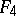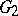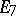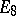# Lie algebra, exceptional

(diff) ← Older revision | Latest revision (diff) | Newer revision → (diff)
A simple Lie algebra (see Lie algebra, semi-simple) that is not classical. Over an algebraically closed field of characteristic zero there are 5 exceptional Lie algebras:,,,, and, of dimension 78, 133, 248, 52, and 14, respectively. The indices are the ranks of these Lie algebras. The simplest linear representations of these exceptional Lie algebras have dimensions 27, 56, 248, 26, and 7, respectively. The algebrais the algebra of derivations (cf. Derivation in a ring) of the Cayley–Dickson algebra andis the algebra of derivations of the unique exceptional Jordan algebra, which can be represented as the algebra of Hermitian matrices of order three over the Cayley–Dickson algebra. The algebrais the linear envelope of the derivations and the multiplications by elements of the exceptional Jordan algebra. The algebrasandand the forms of all the exceptional Lie algebras over algebraically non-closed fields are also connected with the Cayley–Dickson algebra. The various models of exceptional Lie algebras are obtained by considering their gradings by means of cyclic groups (cf. also Graded algebra). The connected Lie groups corresponding to the exceptional Lie algebras, called exceptional groups, are often denoted by the same letters. For example, the complex groupis the group of automorphisms of the Cayley–Dickson algebra over; the complex groupis the group of automorphisms of the exceptional Jordan algebra over.